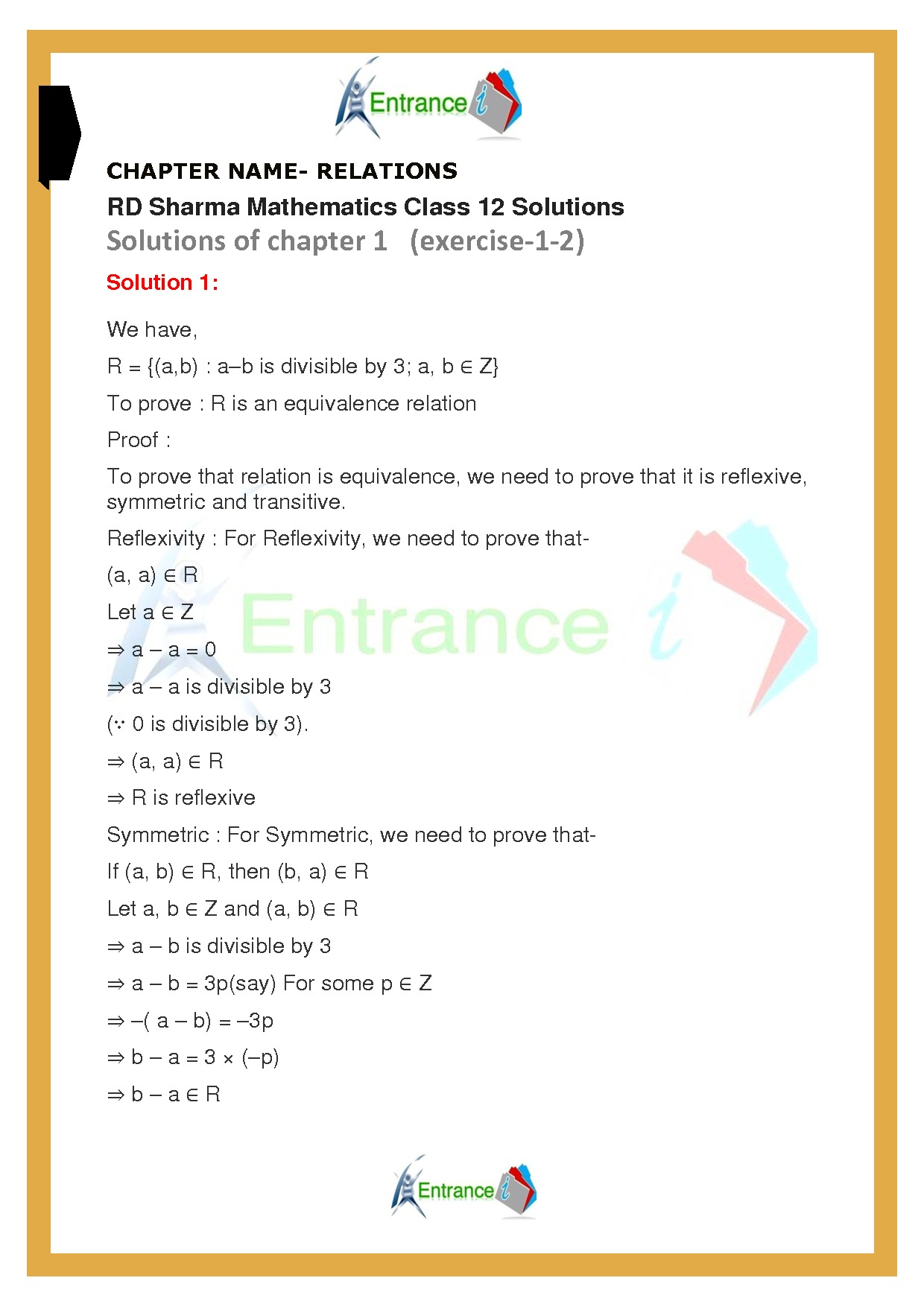# RD Sharma solutions for class 12 maths chapter-1 Exercise-1B

## RD Sharma solutions for class 12 maths chapter-1 Exercise-1B

Download chapter-1 Relations of RD Sharma text book for class 12 maths. All questions of exercise-1B are solved by expert’s faculty members.

Do read the maths formulas prepared by entrancei.

### Download free pdf of RD Sharma solutions for class 12 maths chapter-1 Exercise-1B